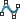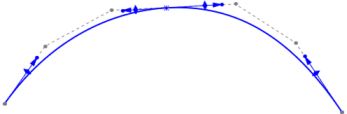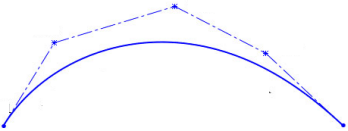Home » How to create Splines in SolidWorks?

# How to create Splines in SolidWorks?

## What is Spline?

A spline is a mathematical curve. It is a type of piecewise polynomial function. Spline curves are also used in computer graphics and computer-aided design (CAD) to approximate complex shapes. There are different ways by which we can create the splines in Solidworks. A spline is a smooth curve that passes through the points in the CAD. This feature is available in both 2D and 3D sketching.

Options available in Solidworks to create the splines are-

• Spline
• Style Spline• Equation Driven Spline

### Crating Spline

To create a spline go to Sketch > Splines icon.In a simple Spline, we have to place the first point on the plane or surface/face to start the spline. Place the second point, third, and likewise according to the need. After placing the first point the curve start to create which takes a shape of a smooth curve passing through these points. We can also say that the curve line is tangent to the point.

See also  How to Become a Certified SolidWorks Associate: A Step-by-Step GuideStyle Splines are a good option when it is important to have a smooth curve. We can define and control the curves using control vertices. There are no through points, so a style spline has only one span between the endpoints. You can infer style splines for tangency or equal curvature. You can constrain the points and dimension the curves’ sides. These curves also support mirroring and self-symmetry.In Equation Driven Spline we have to create the equations to create the spline. You can give an Explicit equation or parametric equation as well to create the curve.

### Spline handle

If you notice after creating Splines at each point you will see arrows. At each end, you will see only one arrow and at the points, in the middle, you will see two arrows. These arrows are called handles. You can define and modify them using several controls, including spline points, spline handles, and control polygons. You can apply curvature constraints at each endpoint. At each through point, you can weigh the tangency vector and control the tangency direction.Using Spline point you can change both size and angle of the handle. From the arrow on the handle you can change only the size and form one shape on the handle you can change only the angle.

### Giving relation and constraints to the spline handle

To add constraints to the handle click on the handle and select the constraints to form the property manager. Just like we give constraints to the line we can give constraints to the spline handles as well. We can draw or use the sketches to give relations and constraints.### Give dimensions to the handle

Just like we give dimensions to the sketch in the same way we can give dimensions to the handle.